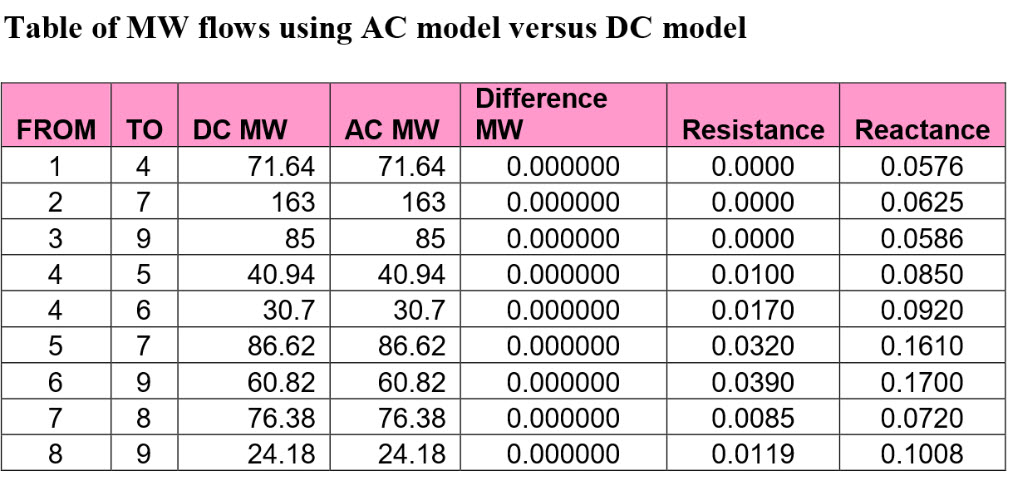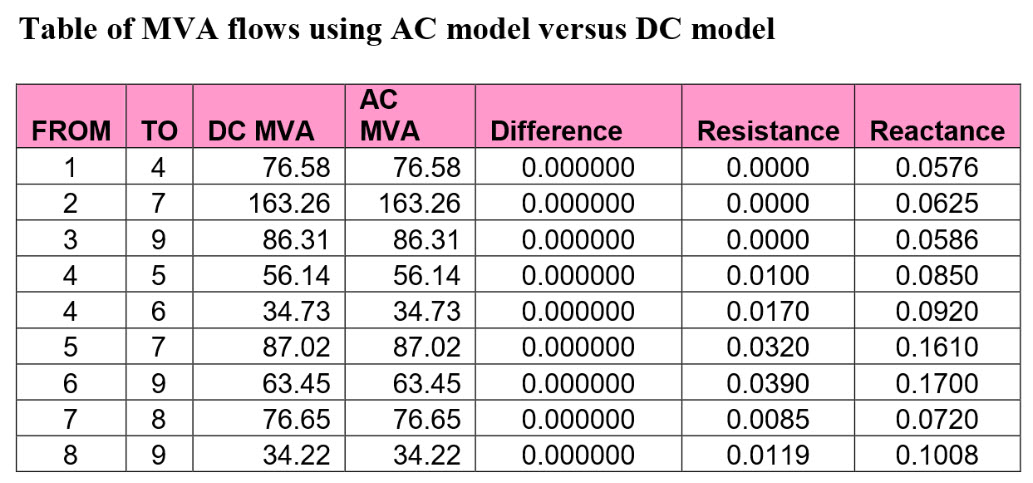# Generation Shift Factors (GSF)

## AC vs DC Power Flow Methods

The project required the computation of the generation shift factors (GSF) based on a defined generator bus outage using the fast-decoupled XB version. The GSF values were used to approximate the post-contingency branch flows based on a pre-contingency branch flow and the generator output before the contingency. The AC power flows were compared with the DC method for the MW and MVA flows. Source code is provided in the same WinZip file for the functions calculating the generation shift factors and the approximated post-contingency branch flows.

## My Summary:

My conclusions will summarize my initial approaches to the project, the results, and opportunities for improving deficiencies. I started the program flow by writing code to determine the swing bus, since it plays a valuable part in the calculation of generation shift factors, and reducing the B prime matrix. I authored my own code to generate the B-prime matrix based on the standard algorithms found in most power systems analysis book. However, I did not originally consider any affect on shunt capacitors. Actually, since the problem was defined for a fast-decoupled solution, I remembered from the professor’s first lecture that shunt reactors were assumed ignored in the model. Before the class discussion on 09-15-2005, I failed to find the documentation for the “makeB()” function. I later included the code to utilize the function as a check to my own code. Since they were both fast-decoupled, the program produced identical matrices. I was originally confused as to how to calculate the P and Q branch flows, but from the class discussion it was assumed that the branch flows would be taken from the “From” column of the power flow branch flows matrix. The P,Q, results will be printed in a nice columnar format when the “project1.m” file is run.

The second portion of my project program was the contingency analysis. The generator bus outage was defined in the code as a variable. For future projects, I could call a user input to set the variable(s), (and may include the possibility of more than one generator being out-of-service). The main program will then print which generator is being outages and then make a call the computeGSF() function where the generation shift factors for each branch are computed, returned to the workspace, and printed in a nice table in the MATLAB command window. The remaining portion of the program determined the post-contingency branch flows. The determineBranchFlows() function was called, returned results to the workspace, and printed the estimated MW flows on each branch based on a specific generator being out of service.

## Demos

The demonstration of Matlab code, output, and tables are discussed in the project report that you can download in PDF format here.## My Solution Code